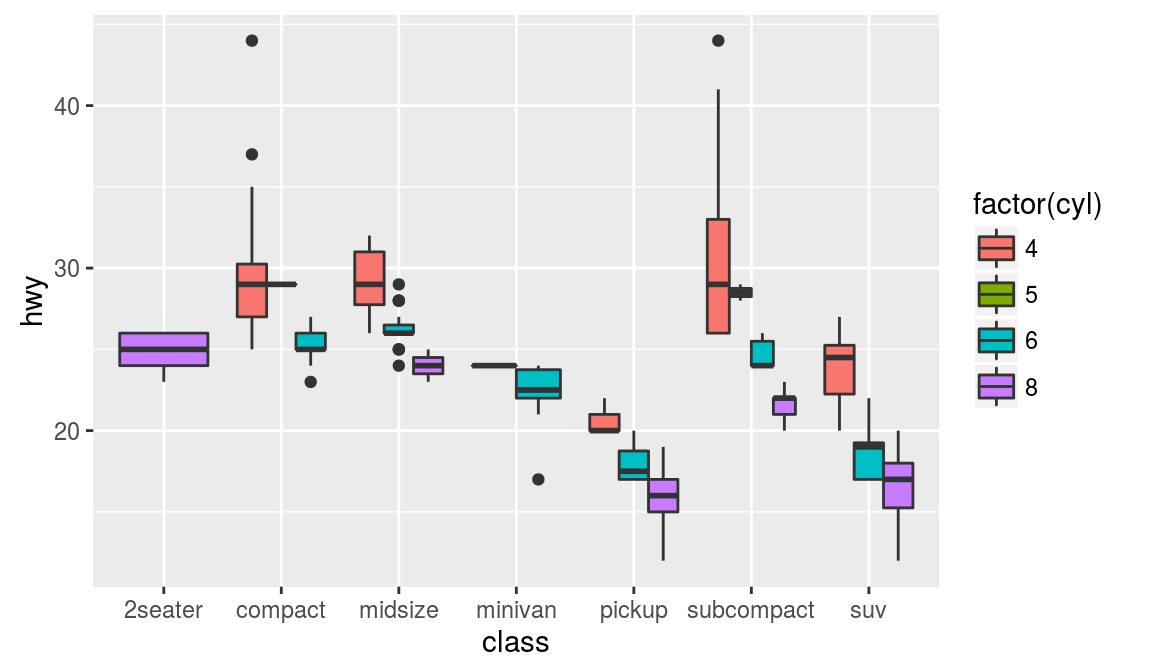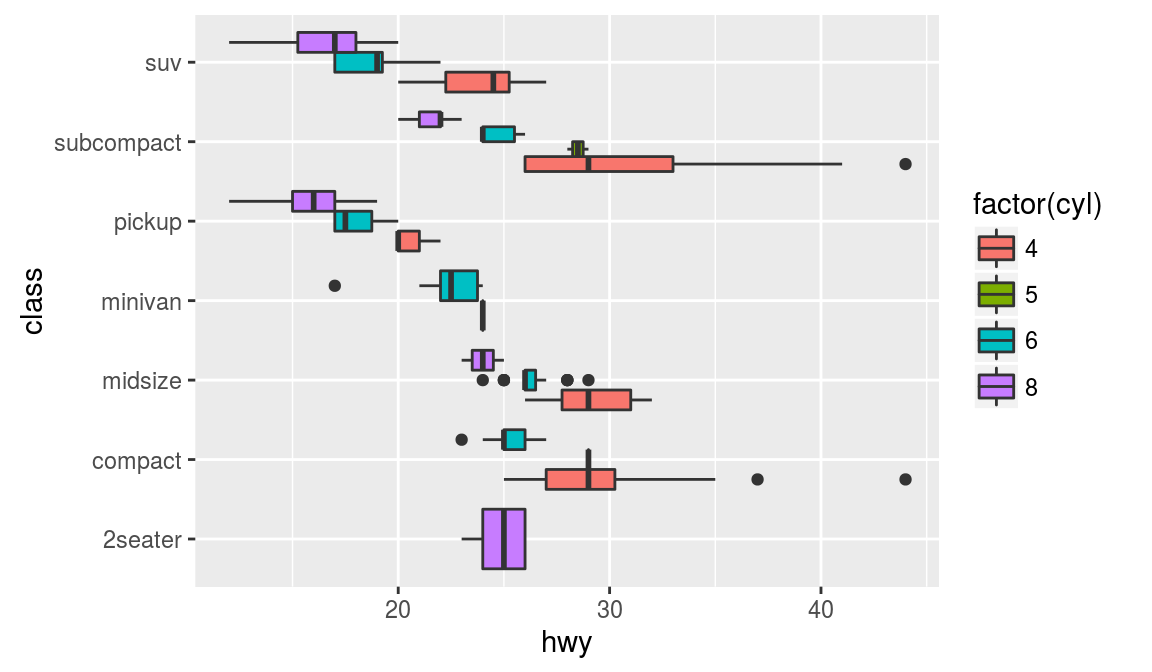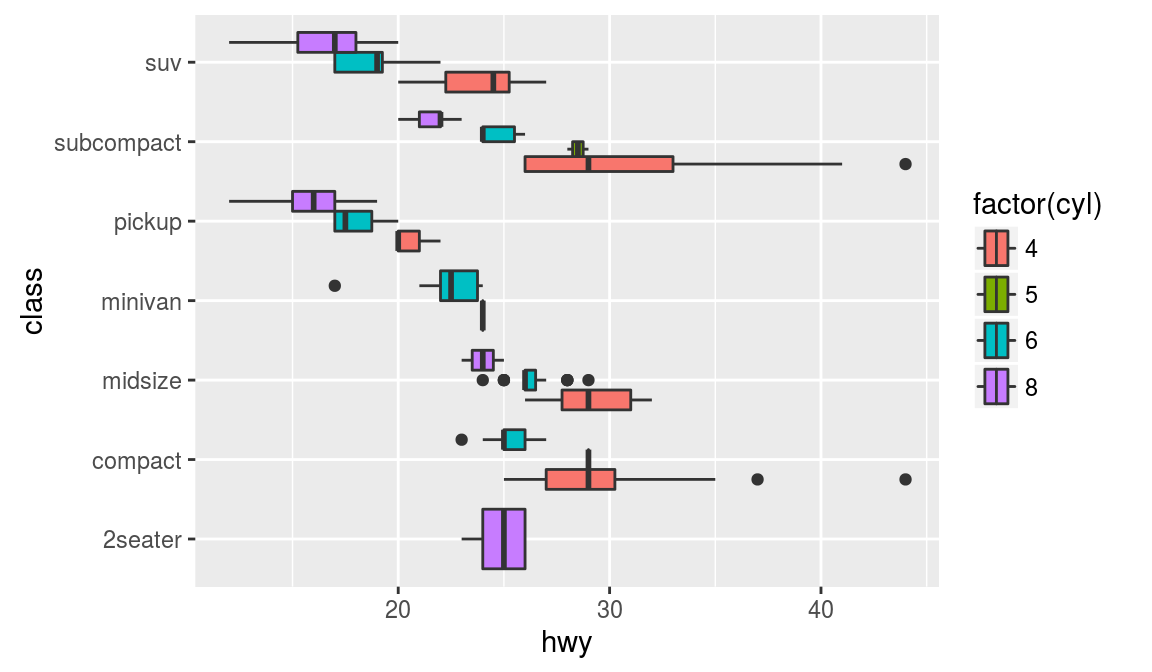ggplot2 now has an official extension mechanism. This means that others can now easily create their own stats, geoms and positions, and provide them in other packages. This should allow the ggplot2 community to flourish, even as less development work happens in ggplot2 itself. This page showcases these extensions.

### ggstance

https://github.com/lionel-/ggstance

ggstance implements horizontal versions of common ggplot2 geoms.

``````# Example from https://github.com/lionel-/ggstance
library(ggplot2)
library(ggstance)

# Vertical
ggplot(mpg, aes(class, hwy, fill = factor(cyl))) +
geom_boxplot()````````````# Horizontal with coord_flip()
ggplot(mpg, aes(class, hwy, fill = factor(cyl))) +
geom_boxplot() +
coord_flip()````````````# In ggstance, you supply aesthetics in their natural order:
# Horizontal with ggstance
ggplot(mpg, aes(hwy, class, fill = factor(cyl))) +
geom_boxploth()``````